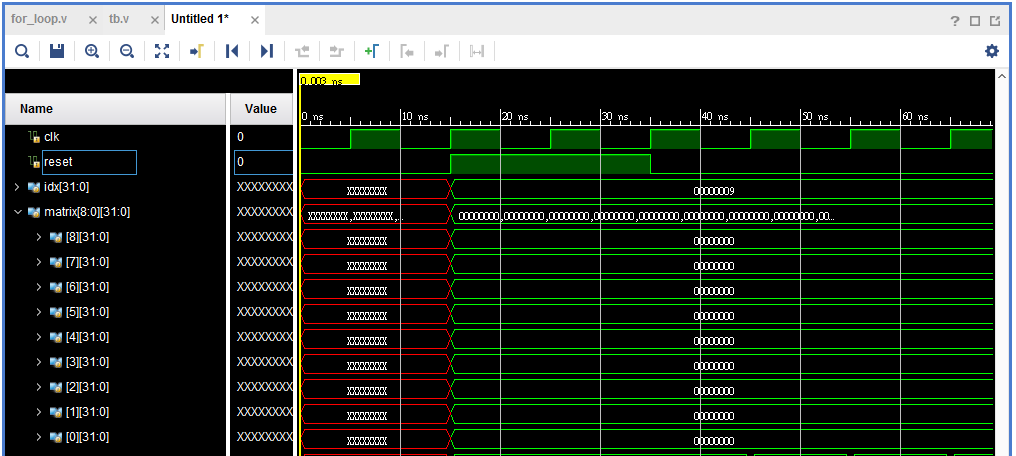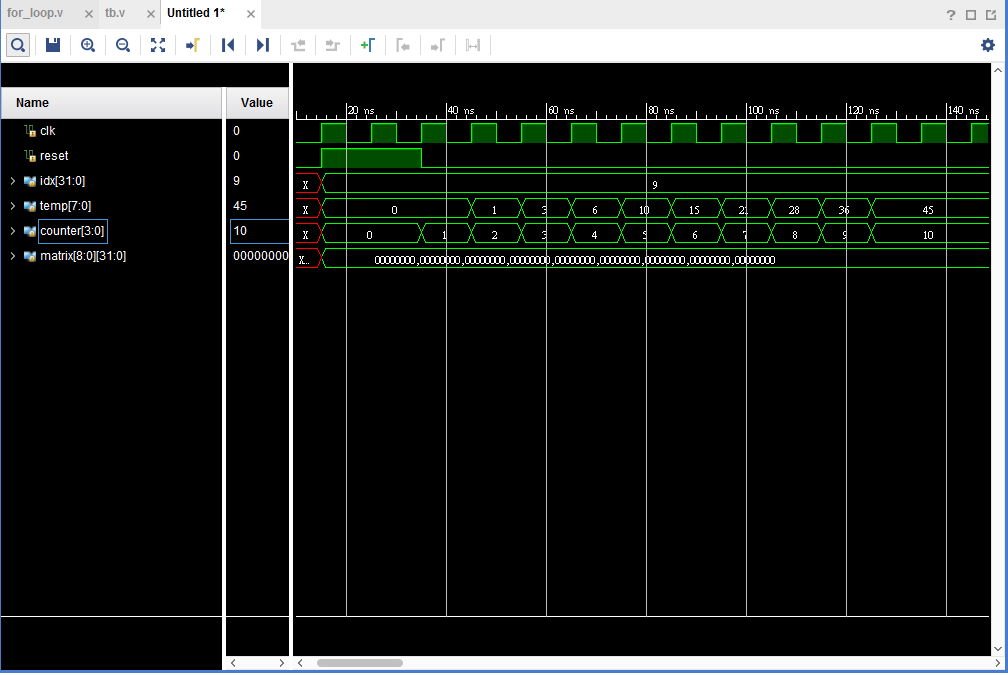#2018 iT 邦幫忙鐵人賽DAY 6
2

## [Day6]for loop

Verilog:

``````reg[31:0]matrix[8:0];
always@(posedge clk)begin
if(reset)
for(idx=0; idx <9; idx = idx +1)begin
matrix[idx] <= 0;
end
end
``````

C code:

``````int matrix;
for(idx=0;idx<9;idx++){
matrix[idx] = 0;
}
``````

``````always@(posedge clk)begin
matrix <= 0;
matrix <= 0;
matrix <= 0;
matrix <= 0;
matrix <= 0;
matrix <= 0;
matrix <= 0;
matrix <= 0;
matrix <= 0;
matrix <= 0;
end
````````````reg [7:0]temp;
always@(posedge clk)begin
for(idx=0;idx<10;idx=idx+1)begin
temp <= temp+idx;
end
end
``````

``````always@(posedge clk)begin
temp <= temp+0;
temp <= temp+1;
temp <= temp+2;
temp <= temp+3;
.
.
.
temp <= temp+9;
end
``````

``````reg[3:0]counter;
always@(posedge clk)begin
if(reset)
counter <= 0;
else
counter <= (counter == 10) ? counter : counter  + 1;
end

always@(posedge clk)begin
if(reset)
temp <= 0;
else if(counter<10)
temp <= temp + counter;
end
``````Verilog 從放棄到有趣30